Select Page

# CBSE Maths 12 Science MCQ Vector Algebra Solutions in English

CBSE Maths 12 Science MCQ Vector Algebra Solutions in English to enable students to get Solutions in a narrative video format for the specific question.

Expert Teacher provides CBSE Maths 12 Science MCQ Vector Algebra Solutions through Video Solutions in English language. This video solution will be useful for students to understand how to write an answer in exam in order to score more marks. This teacher uses a narrative style for a question from Vector Algebra not only to explain the proper method of answering question, but deriving right answer too.

Please find the question below and view the Solution in a narrative video format.

Question:

Solution Video in English:

You can select video Solutions from other languages also. Please check Solutions in ( Hindi )

## Similar Questions from CBSE, 12th Science, Maths, Vector Algebra

Question 1 : If the vertices A, B, C of a triangle ABC have position vectors (1, 2, 3), (-1, 0, 0), (0, 1, 2) respectively then find <ABC (<ABC is the angle between the vectors BA and BC). (View Answer Video)

Question 2 : Find |x|, if for a unit vector a,(x - a).(x + a) = 12. (View Answer Video)

Question 3 : Find a vector in the direction of vector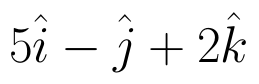which has magnitude 8 unit. (View Answer Video)

Question 4 : Find the scalar and vector components of the vector with initial point (2, 1) and terminal point (-5, 7). (View Answer Video)

Question 5 : For given vectors,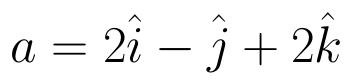and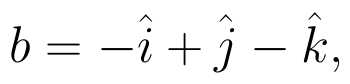find the unit vector in the direction of the vector a + b. (View Answer Video)

### Relations and Functions

Question 1 :  Letdefined as f(x) = 5 be a constant function. Then its range is (View Answer Video)

Question 2 : If f and g two functions such that they are one-one then g o f is: (View Answer Video)

Question 3 :  If f(x) = ax + b and g(x) = cx + d, then f[g(x)] – g[f(x)] is equivalent to, (View Answer Video)

Question 4 : If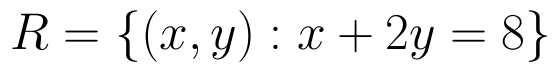is a relation on N, write the range of R. (View Answer Video)

Question 5 : Let A = {1, 2, 3, 4} and let R = {(2, 2), (3, 3), (4, 4), (1, 2)} be a relation on A. Then, R is, (View Answer Video)

### Three Dimensional Geometry

Question 1 : Find the co-ordinates of the point where the line through the points A(3, 4, 1) and B(5, 1, 6) crosses the XY-plane. Also find angle which this line makes with the XZ-plane. (View Answer Video)

Question 2 : Write the distance between the parallel planes 2x - y + 3z = 4 and 2x - y + 3z = 18. (View Answer Video)

Question 3 : Find the distance of the point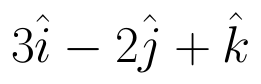from the plane 3x + y - z + 2+0 measured parallel to the line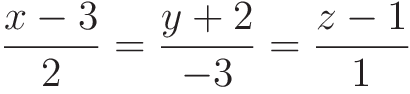Also, find the foot of the perpendicular from the given point upon the given plane. (View Answer Video)

Question 4 : Find the distance of the point (-1, -5, -10) from the point of intersection of the line joining the points A(2, -1, 2) and B(5, 3, 4) with the plane x - y + z = 5. (View Answer Video)

Question 5 : If a line has the direction ratios -18, 12, -4, then what are its direction cosines? (View Answer Video)

### Application of Integrals

Question 1 : Using the method of integration find the area of the triangle ABC, coordinates of whose vertices are A(2, 0), B(4, 5) and C(6, 3). (View Answer Video)

Question 2 : Using the method of integration find the area bounded by the curve |x| + |y| = 1. (View Answer Video)

Question 3 : Using the method of integration, find the area of the region bounded by the lines 3x - 2y + 1 = 0, 2x + 3y -21 = 0 and x - 5y + 9 = 0. (View Answer Video)

Question 4 : Given that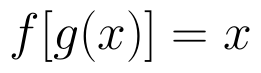, for x = 0 to x = 20. Find f(x) and g(x) such that the area between f(x) and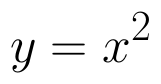from x = 0 to x = 5 be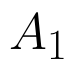and area between g(x) and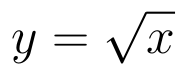from y = 0 to y = 5 be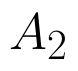. Is? Like functions f and g which work is better, team work or individual work? (View Answer Video)

Question 5 : Find the area bounded by the curve y = sin x between x = 0 and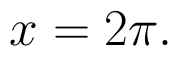(View Answer Video)